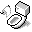LaTeX

In the examples section, the <tex> code used to generate the formulas is listed first, followed by the rendered graphic, and finally some quick comments about the commands used.

Note that Puchu.Net uses a utility called mimeTeX, and it has some differences from full TeX/LaTeX implementations.

## References

Resources on how to work with LaTeX commands and syntax.

## Examples

<tex>\mathbf{p} = (p_{x}, p_{y}, p_{z}, p_{w})</tex>\mathbf{?} changes to bold typeface, _{?} creates subscript.

<tex>\mathbf{p} = ( \frac{p_{x}}{p_{w}}, \frac{p_{y}}{p_{w}}, \frac{p_{z}}{p_{w}}, 1)</tex>\frac{?}{?} creates fractions.

<tex>\mathbf{M_{4\times4}}=\left(\begin{array}{cccc} m_{00} & m_{01} & m_{02} & 0 \\ m_{10} & m_{11} & m_{12} & 0 \\ m_{20} & m_{21} & m_{22} & 0 \\ 0 & 0 & 0 & 1 \\ \end{array}\right)</tex>\times creates the multiply symbol as seen in; \begin{array} and \end{array} indicate start and end of array, each row is delimited by \\, each column is separated by &. \left( and \right) create the parentheses. The {cccc} specifies, for each column, the alignment for the elements; c is centered, l is left-aligned, and r is right-aligned.Document is accessible from http://www.puchu.net. © 2002-2010 Sean Yang, Karen Yang, Don Yang and/or respective authors, all rights reserverd. This material may contain (biased) opinions, inappropriate materials for numerous individuals, work of other authors from the internet, links that refer to other web documents and resources, or origial work that cannot be use for personal or commercial purposes. Please respect the work of original authors.